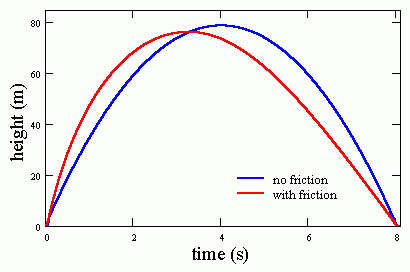## How high did it go?

When launching rockets, we like to know how high they go. There are two existing ways to measure this: (1) Put an expensive altimeter on the rocket and hope it survives the next crash, or (2) use trigonometric data from one or more remote observers with inclinometers and compasses. Here I propose a third method that requires only one remote observer with a stopwatch.

The new method to calculate apogee depends on a mathematical model, described below, for rocket flight following the thrust phase. While the development of the model uses calculus-based classical physics, knowledge of this is not required to use the results of the model. I provide a graphical technique and a simple equation that allow people with less mathematical experience to calculate rocket apogee heights.

Also note that the results here apply to ballistic objects other than water rockets. With a stopwatch I have used the method to estimate how high I can kick a ball (answer: not too high).

## The thrust phase

One of the distinguishing features of water rockets is that the thrust phase of the flight takes place so quickly. Typically it takes less than 0.1 second to expel all the water, and perhaps another 0.05 second to expel the remaining high-pressure air. Once thrust is finished, a certain amount of time will have elapsed since “lift off,” and the rocket will be at a particular height above the ground and have a particular velocity. I will designate these respective “burnout” quantities as

t0h0v0.

The main purpose of water-rocket flight simulators, as I see it, is to get an accurate v0, which depends on things such as starting pressure, amount of water, nozzle shape, volume of bottle, etc. In general the other burnout quantities, t0 and h0, are not terribly significant in the ensuing calculations due to their smallness. Reasonable approximations for most purposes are

h0 = 3 m,  t0 = 2h0/v0.

## The coasting phase

For the remainder of the flight after the thrust phase, Newton‘s law give the following equation for rocket motion:where m is the mass of the (empty) rocket, a is its acceleration, and the Fs are the forces on the rocket. Assuming vertical (or nearly vertical) subsonic flight we make the substitutions;

where v is vertical velocity, g is acceleration due to gravity (around 9.81 m/s2),is the density of the external air (around 1.18 kg/m3), A is the frontal or cross-sectional area of the rocket, and CD is the coefficient of drag. CD depends on the shape of the rocket, but (we hope during normal flight conditions) is largely insensitive toand v. The drag force changes sign during flight: it is negative (downward acting) during ascent and positive (upward acting) during descent. Putting these substitutions into the equation of motion and dividing by mg we obtain the differential equation,

where vt is the terminal velocity of the rocket (the steady-state freefall velocity) given by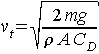.

To solve the differential equation, we rearrange a few things and integrate both sides:.

It should be noted that the mathematical development here allows for vt to change from one value to another one at (or near) the point of apogee. For instance, if at apogee a parachute is deployed or mass is ejected or the rocket destabilizes and falls sideways, vt will have one value for ascent and another (smaller) value for descent. I use the distinct quantities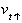and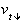to account for this possibility. Conversely, a ballistic flight—as I define it—is one in which the rocket has a single terminal velocity,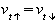. This is known also as a lawn-dart or scud-style flight.

## The solution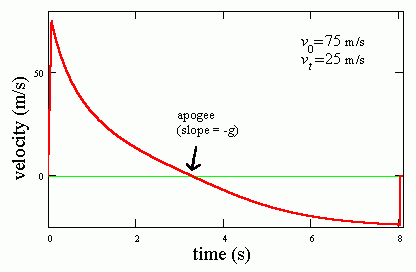This graph shows a ballistic velocity vs. time curve for a typical 2-liter water rocket. The curve is generated by the solution to our equation of motion, namely,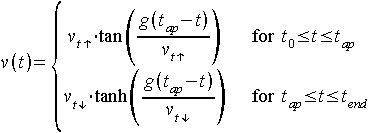where tap is the elapsed time at apogee and is given byand tend is the time of touchdown (or impact). Note that the trigonometric functions used here must be in terms of radians rather than degrees.

To get height as a function of time, we substitute v = dh/dt and integrate once more using our initial conditions. After some algebra the result iswhere the apogee height hap is given by.

Assuming that touchdown occurs at the same elevation as the launch (h = 0), the velocity and elapsed time at that point are.

## Graphical results

The above relations can prove useful in the field, away from simulation programs and spreadsheets, provided they are given in graphical form. Here is a 207KB PDF (AdobeAcrobat) file which can be printed onto a single sheet of paper. The graph gives the correlation between five quantities:

1. Terminal velocity [vt] - for ballistic flights this value applies during the entire flight. For recovery-deployment flights this will be the value during ascent only. In both cases this value should not vary from flight to flight, that is, it only needs to be determined once for a given rocket.
2. Burnout velocity [v0] - a quantity which varies from flight to flight unless the amount of water and pressure are fixed carefully to particular values.
3. Apogee height [hap] - can be independently measured with an altitude sensor in the rocket or by use of an inclinometer or theodolite.
4. Time to apogee [tap] - should be able to collect this value for every rocket flight. (Remember it is easier to judge the point of apogee if you are standing reasonably far away from the launcher.)
5. Time difference [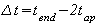] - can collect this value only during a ballistic flight. You can collect both tap and tend during the flight if you use a stopwatch with a multiple-lap storing feature (most digital wristwatches have this) or you use a video camera to record the flight for later analysis.

Here is the key: you only need to know two of the five in order to solve for the remaining three quantities. This means that carefully recorded flight times for a ballistic flight (no recovery mechanism) can give you the terminal velocity, the burnout velocity, and apogee height.

Some people believe that this graphical method does not work if your rocket deploys a parachute. This is not true. The method only requires that you first obtain vt for the parachute-deploying rocket by some means. The means could be to

• launch the rocket once without a parachute and measure tap and tend
• estimate CD based on experience (knowing CD is equivalent to knowing vt)
• measure tap and hap (using trigonometry) for one parachute-deploying flight.
Having determined vt for the rocket, time to apogee tap is all that is needed on subsequent flights to calculate the apogee height.

The use of the graph is illustrated by an example below.

Example: A rocket with no recovery mechanism is launched. Time-to-apogee is 3.24 seconds and total time to impact is 7.96 seconds. This gives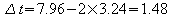. We locate the point on the graph where the intersection of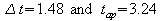occurs. From this point we can determine that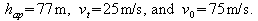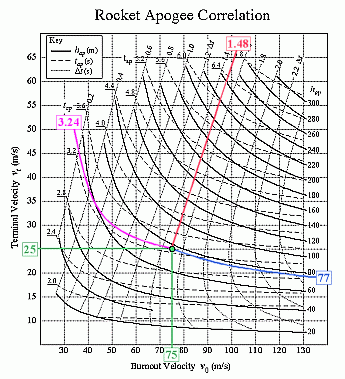## Assumptions

There are two main assumptions in this analysis. Let us reexamine them:

1. We assumed that the coefficient of drag is constant. In reality, it depends somewhat on velocity—normally CD decreases as velocity increases. This means that the terminal velocity and hence CD obtained by my method will be an average value over a range of velocities. Consequently, the apogee heights and burnout velocities that are calculated from the time quantities may be overestimated by a few percent.
2. We assumed that the flight is purely vertical. Of course, no flight is purely vertical due to wind currents and non-vertical launch angles. However, the error in a calculated apogee is less than a couple percent as long as the launch angle is within 10 degrees from vertical. My method can get satisfactory results without the kind of careful corrections for wind and nonvertical trajectories that the trigonometric methods require.

## The simplest correlation

It turns out that for ballistic flights, there is a simple empirical correlation that can give quite accurate apogee heights from total flight times:

hap =  0.122 g (tend)2 ,

where hap and tend are in units of meters and seconds, respectively, and g = 9.81 m/s2. If you have any doubts about the correlation, compare its predictions to those of any decent water-rocket simulator, or to your own in-the-field measurements—you may be surprised how well it does over a wide range of conditions.

The above equation is very closely related to an equation known as Littlewood's law:

hap = (g/8)(tend)2 ,

named for mathematician J.E. Littlewood, who did some artillery-related math for the British military during World War I. This “law” is actually an approximation based on the fact that if a frictionless ballistic flight and a “real” flight have the same total flight time, they will also have nearly the same apogee height.

The graph below compares a typical rocket flight to an ideal frictionless flight where both have the same total flight time. The two flights have a correspondingly similar apogee height—note, however, that the two flights do not have the same burnout (initial) velocities as given by the slope of the curve at very small times.